• Call Now

1800-102-2727•

# Law of conservation of energy - definition, examples, Practice problems, FAQs

Energy is everywhere. Can you imagine running smartphones,laptops, fans etc without electrical energy? Can bicycles, gym equipment and lawn mowers function without mechanical energy ? Starting from micro appliances like toy cars to huge power plants, energy is everywhere. Sun is considered the crux where all energy comes from and we get the energy for our life to sustain. Solar energy is the oldest form of energy known to man. In physics, energy needs to be quantified. It can neither be created nor destroyed, but can only be transformed from one form to another. The term “energy” was first used by British scientist Thomas Young, in 1802. Conservation of energy has many real life examples: chemical energy consumed by us as food and it is then used in doing mechanical work. Electrical energy is consumed by a bulb and then it is dissipated as heat and light. In this article, let us explore energy conservation in detail.

• Definition of energy
• Law of conservation of energy- definition, examples
• Practice problems
• FAQs

## Definition of energy

Energy can be defined as the quantitative property of a body’s or system's ability to do work. It exists in different forms- kinetic, gravitational potential, spring potential, electrical, thermal and so on. The SI unit of energy is Joule (J) named after James Prescott Joule. It carries a dimensional formula of $\left[M{L}^{2}{T}^{-2}\right].$

Note:

The CGS unit of energy is ergs.

Energy also carries other units like British Thermal Units (BTU), Kilowatt Hours (kWh) and calories (cal).

## Law of conservation of energy- definition, examples

Being one of the basic laws in physics, the law of conservation of energy states that for a system isolated from its surroundings, the total energy of the system is always conserved.

For instance, the chemical energy of the petrol at the fuel station is converted into mechanical energy by the car that burns the petrol inside its internal combustion engine, some transforms into heat energy which comes out from the exhaust and warms up the car’s body. Some part also transforms into sound energy as well. Here the petrol pump, the car with its engine and exhaust and the surrounding all constitute an isolated system.The energy possessed by a body by virtue of its position or orientation is called Potential energy (PE). For a body of mass m, lying at a height h above the ground, the gravitational PE can be written as

PE=mgh

Kinetic energy(KE) is defined as the energy possessed by it due to its state of motion.

$KE=\frac{1}{2}m{v}^{2}$

PE and KE are collectively called mechanical energy. When only conservative forces are acting, the change in PE can be written as

${U}_{f}-{U}_{i}=-\int dW=-{\int }_{i}^{f}\stackrel{\to }{F}.d\stackrel{\to }{r}$ ---(i)

Where, Uf is the Final PE and Ui is the Initial PE.

ifF.dr represents the dot product of force acting on the body and the displacement it undergoes, which is nothing but the work done on the body. Now according to the Work Energy theorem , the change in KE of the body is equal to the work done.

i.e., ${K}_{f}-{K}_{i}=W={\int }_{i}^{f}\stackrel{\to }{F.}\stackrel{\to }{dr}---\left(ii\right)$

Kf- Final KE

Ki- Initial KE

From (i) and (ii) we get,

${-\left(U}_{f}-{U}_{i}\right)={K}_{f}-{K}_{i}⇒{K}_{i}+{U}_{i}={K}_{f}+{U}_{f}$

The sum of the total mechanical energy remains constant. This is called the law of conservation of energy.

A ball placed at a height h above the building has purely potential energy when it is at rest. But as it starts falling, its energy becomes kinetic. This is an example of potential energy being converted into kinetic energy.

Let us consider a spring pulled with a force F. It undergoes elongation xp. Now a restoring force

Fs acts in the opposite direction and tries to bring the spring back to its original position. In this position, the energy stored in the spring is $\frac{1}{2}k{x}_{p}^{2}$.

k- stiffness constant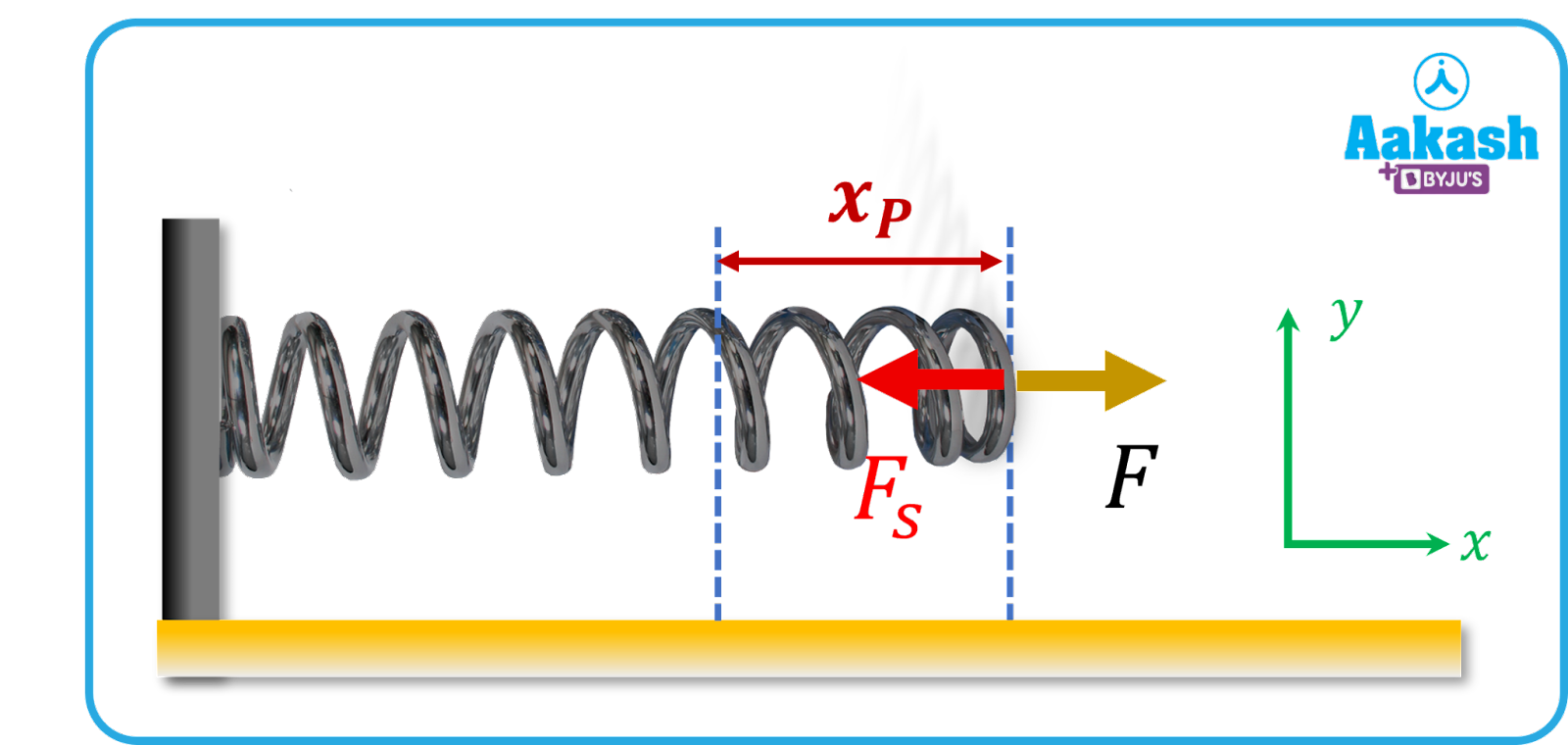When a block of mass M is suspended at the bottom of a spring, the spring elongates by a distance x. The decrease in potential energy of the block is equal to the potential energy stored in the spring.

i.e., $Mgx=\frac{1}{2}k{x}^{2}$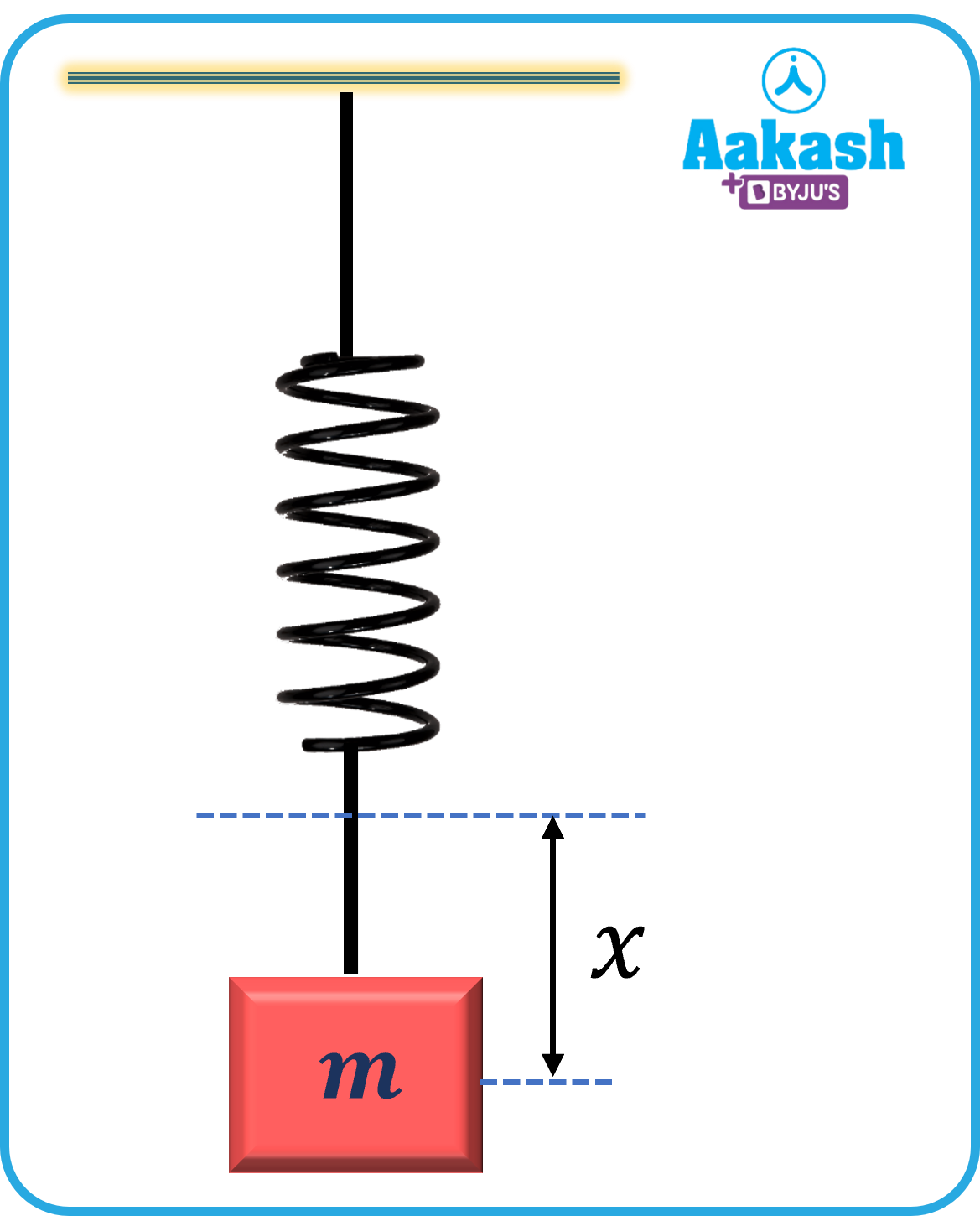Consider a ball of mass m sliding with a velocity v. It strikes a spring kept hinged at one end. In an ideal case the kinetic energy of the ball is converted into the potential energy of the spring.

So,$\frac{1}{2}k{x}^{2}=\frac{1}{2}m{v}^{2}$

The compression in the spring when the ball comes to momentarily rest; $x=v\sqrt{\frac{m}{k}}$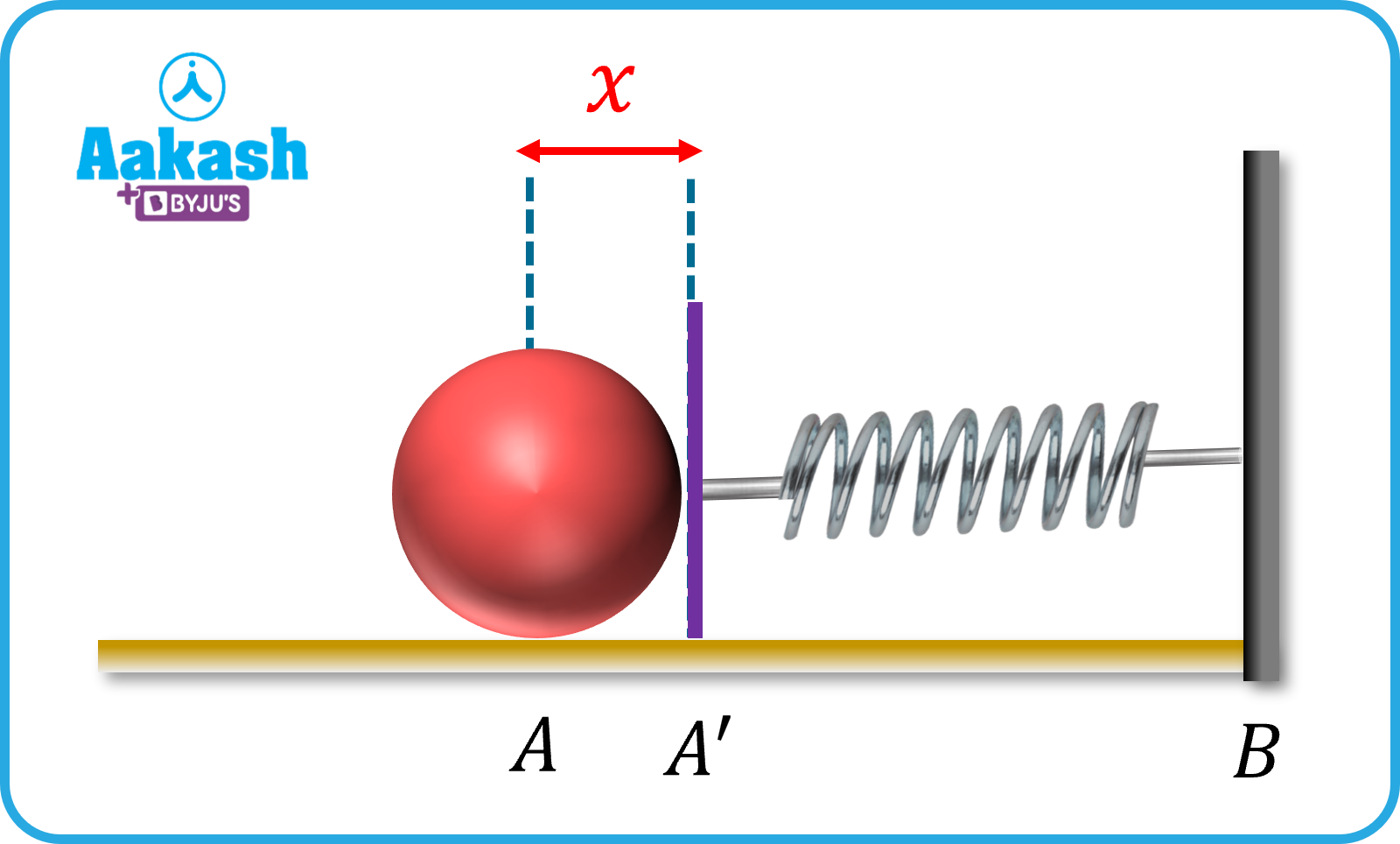## Practice Problems

Q. A body of mass 0.1 kg moving with a velocity of 10 m/s hits a spring (fixed at the other end) of force constant 1000 N/m and comes to rest after compressing the spring. The compression of the spring is

(a)0.1 m (b)0.2 m (c)0.3 m (d)0.5 m

A. a

According to the conservation of energy, the kinetic energy of the ball is converted into spring potential energy.

So, $\frac{1}{2}k{x}^{2}=\frac{1}{2}m{v}^{2}$

Q. The potential energy of a spring when it is stretched by 2 cm is U. Calculate the potential energy stored in the spring when it is stretched by 8 cm

(a)$\frac{U}{4}$ (b)$\frac{U}{2}$ (c)16 U (d)4 U

A. c

Potential energy of a stretched spring where k- stiffness constant of the spring and x is the amount of stretch in the spring.

$⇒\frac{{U}_{1}}{{U}_{2}}=\frac{{x}_{1}^{2}}{{x}_{2}^{2}}$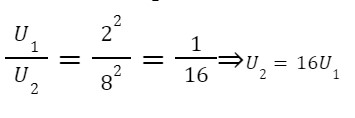Q. In a sports event, an athlete throws a shot put ball having mass 10 kg with a speed of 1 m/s at 450 with the horizontal. He throws the ball from a height 1.5 m above the ground. Consider the air resistance as negligible. The acceleration due to gravity is 10 m/s2. Calculate the kinetic energy of the shot put ball when it reaches the ground.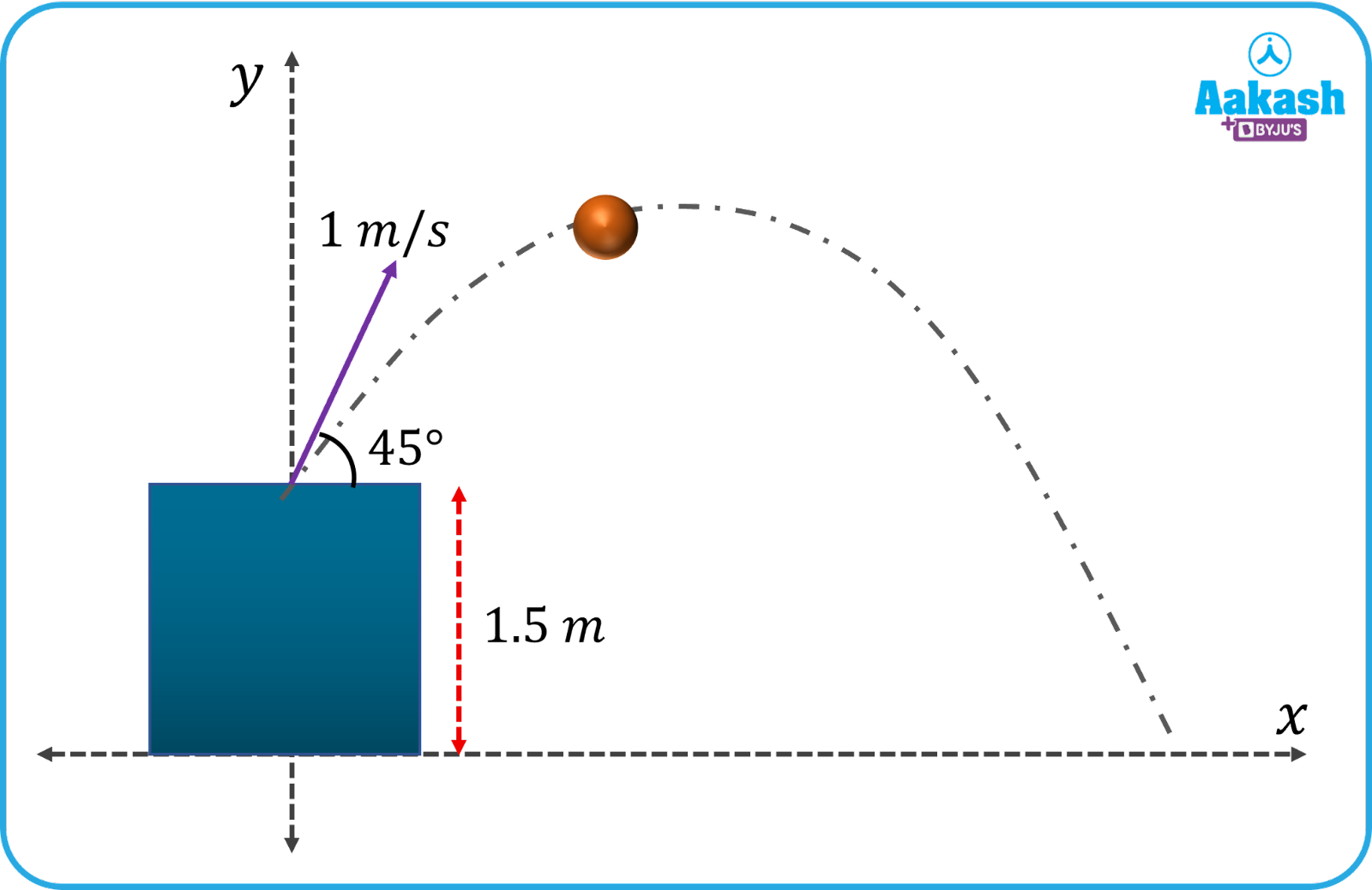(a)100 J (b)155 J (c)120 J (d)100 J

A.Let’s take the reference level for the calculation of PE be the ground.

From the principle of conservation of mechanical energy,

${\left(PE\right)}_{i}+{\left(KE\right)}_{i}={\left(PE\right)}_{f}+{\left(KE\right)}_{f}$

$\frac{1}{2}m{v}^{2}+mgh=0+{\left(KE\right)}_{f}$

Q. Calculate the velocity of the bob of a simple pendulum at its mean position. Given that the bob is able to rise to a vertical height of 10 cm.

(a) 0.6 m/s (b) 1.4 m/s (c) 1.8 m/s (d) 2.2 m/s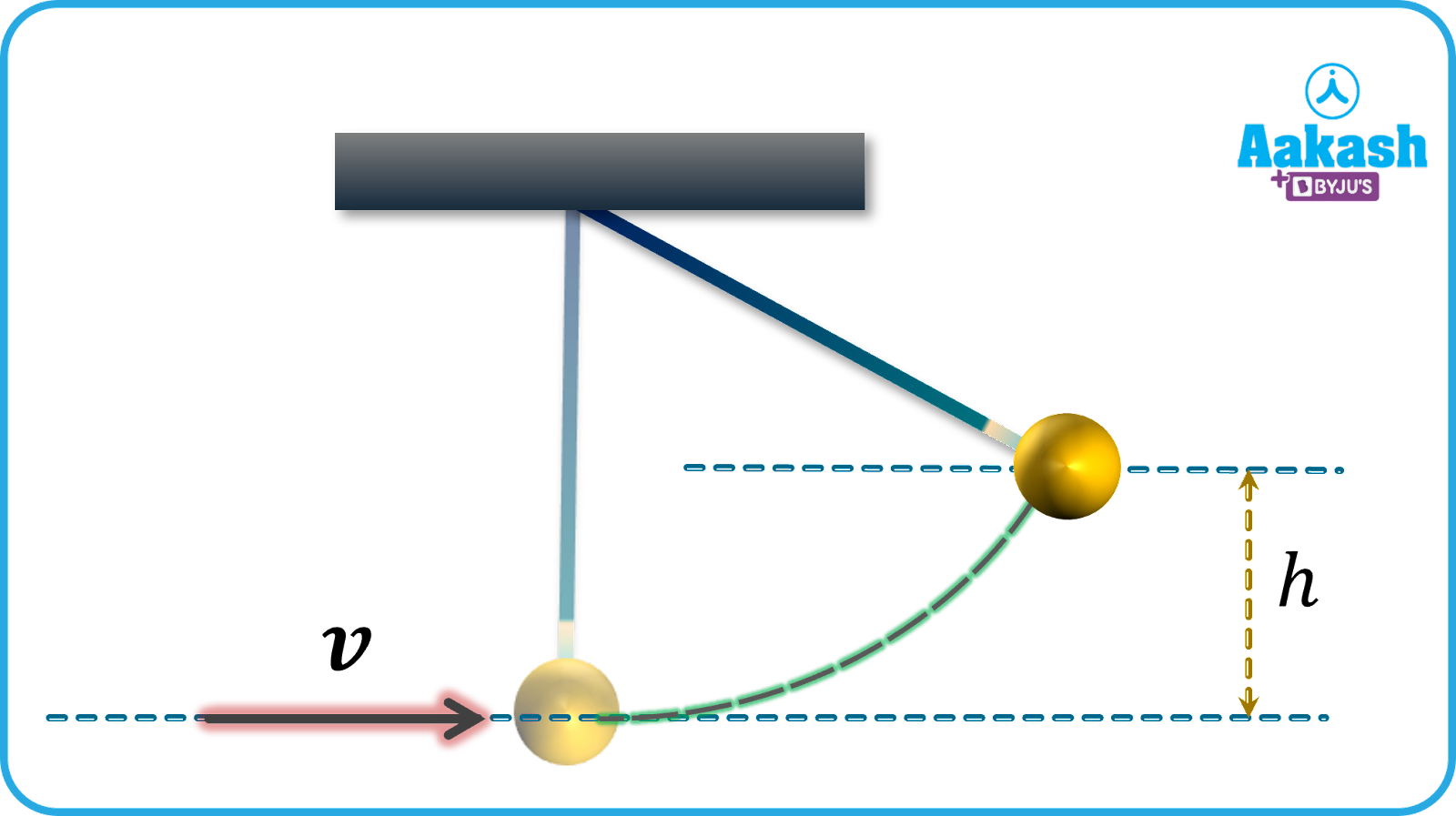A. Increase in potential energy of the ball= decrease in its Kinetic energy

$mgh-0=\frac{1}{2}m{v}^{2}-0$

## FAQs

Q. Where is the law of conservation of energy used in everyday life?
A.
The food we eat supplies chemical energy. This is in turn used for mechanical work when going to the office or school.

Q. Give one example of conversion of chemical energy to mechanical energy.
A.
Fuel burning inside a rocket is used by the rocket engine to create the thrust and helps the rocket to travel up, i.e chemical energy gets converted into mechanical energy.

Q. What is the basic condition for the law of conservation of energy?
A.
The law of conservation of energy holds good unless energy is added from the outside to the system or dissipates out from the system to the surrounding. The only way to hold the conservation of energy is if the system is an isolated system.

Q. Does conservation of energy apply even to the expanding universe?
A.
As the space expands, it releases the stored gravitational potential energy. This gravitational potential energy converts into the intrinsic energy which fills the newly created volume due to the expansion. So we can conclude that the expansion of the universe is also governed by the law of energy conservation.Talk to our expert
Resend OTP Timer =
By submitting up, I agree to receive all the Whatsapp communication on my registered number and Aakash terms and conditions and privacy policy